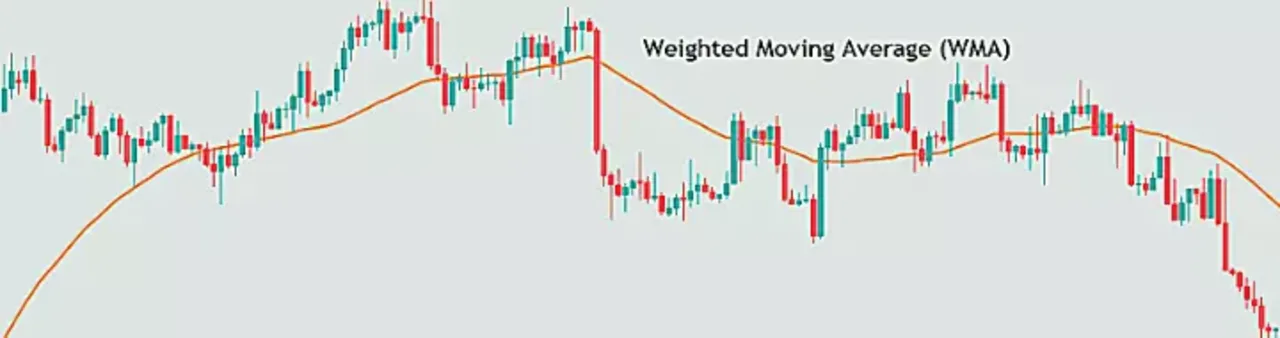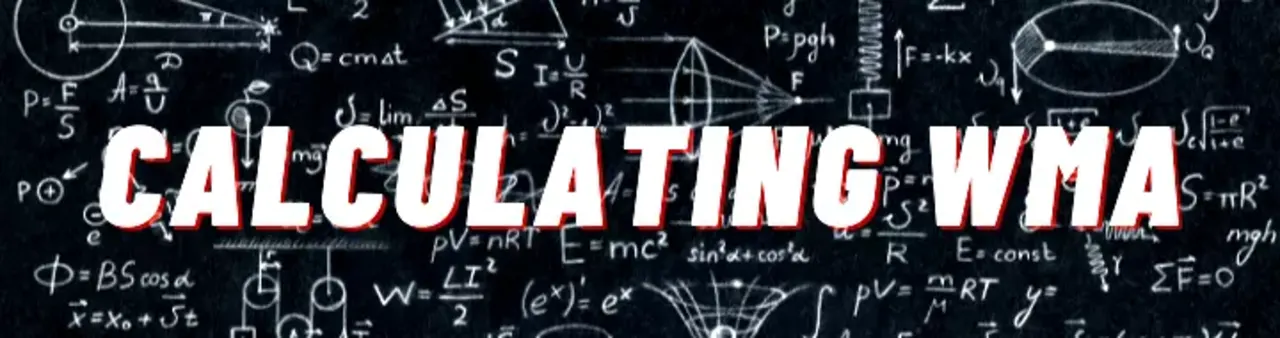# Weighted Moving Average (WMA) - Technical Indicator

Daksh Murkute | | |

I’m sure you know a thing or two about moving averages and might even be using a moving average in trading. But, what if I told you that there is a moving average that you don’t know yet and it might just be a game changer?

In this blog post, I’m going to talk about this type of moving average known as weighted moving average.

Read till the end as I’m not just going to tell you what the weighted moving average is, but will also tell you how it is different from the other moving averages, and also, how you can use it in trading.

### Contents

What is Weighted Moving Average?

How is the WMA calculated?

How is the WMA different from the other moving averages?

How to use the WMA?

Which moving average do you use?

## What is weighted moving average?WMAs are a bit different than the rest of the moving averages out there. Here, each price data gets some weightage in the calculation. The WMA is just like any other moving average. The WMA too, gives us an average of the price over a particular time.

There might be a question as to, how exactly is the WMA different from the other moving averages, and should anyone use this over the others.

The difference lies in the calculation of the WMA. This is what sets it apart from the other moving averages.

Let’ see how the WMA is calculated and then we’ll go on to the part of how exactly the WMA is different from the other moving average.

## How is the WMA calculated?WMA = ( Price * n + Price(1) * n-1 + ... Price( n-1 ) * 1) / ( n * ( n + 1 ) / 2 )

where, n = time period

This formula might give much clarity but you should know one thing that, the WMA assigns different weightage to every price data.

The most recent price data gets the highest weightage and as the price becomes older, the weightage that it gets decreases.

If you see the formula of the WMA, you’ll see that this moving average too calculates an average of the prices only, but the thing that is different here is that the prices are multiplied by the weightage that is to be assigned to each of them.

So if you want to calculate a WMA of 5 periods, then according to the formula, the first price data will have a weightage of 4/10, that is 40%, the second price data 3/10 or 30%, third price data 2/10 or 20%, and the oldest price data will have a weightage of 1/10 or just 10%.

## How is the WMA different from other moving averages?As I said, the WMA is different from other moving averages on the basis of how it works and how it is calculated.

As you already in the calculations part, the WMA gives an additional weightage to the recent price data right, what this does, in turn, is that it makes the WMA react quickly to the price changes.

What this means is that compared to other moving averages WMA will absorb price movement faster and will follow the price very closely.

Another advantage in the WMAs is that, as the price gets older, its weightage decreases gradually, so when the time comes to discard the price from the calculation, it does not disturb the WMA much and the price fades away smoothly.

Now you might say, all this is alright, but how to use the WMAs, let’s get onto that part now.

## How to use the WMA?

You can use the WMA in trading just as you’d use any other moving average. There are no special uses or ways to trade the WMAs.

The WMAs can be used to know the trend. If the price is above the WMA and the WMA is also sloping up, then this is a bullish trend indication.For a bullish trend indication, the price should be below the WMA and the WMA should be sloping down.

The method of moving average crossovers can be used here too. Use two WMAs, one of a smaller length and the other of a longer length.When the smaller length WMA crosses the longer length WMA to the upside, this is bullish, and when it crosses it to the downside, then it is bearish.

Some traders use moving averages as dynamic support and resistance. The same method can be applied to the WMAs too.

You can look for the price trending in a direction, wait for a pullback, and then enter when you get a confirmation that the price is resuming its trend.One thing to note is that, if you see that the WMA is not sloping in any direction but it is moving flat and the price is just hovering around it, you should not use of the methods I’ve mentioned above.

The flat WMA indicates that the price is ranging and you will be better off using some other tools to trade this.

## Which moving average do you use?

Do let me know which moving average do you normally use in trading and also, do let me know if you’ve ever tried out the WMAs.

Share this blog post with others and let them also know about this type of moving average that might be one of the best moving averages out there.

Feel free to reach out through the comments section for absolutely anything and I’ll get back to it at the earliest.